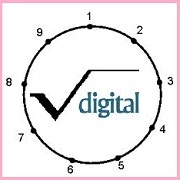# Digital RootThe repeated digital sum, or digital root of 6788 is 2.

6+7+8+8 = 29

2+9 = 11

1+1 = 2.

What is the Digital root of the 180 digit number 1011121314.... ....96979899 made from the numbers 10 to 99 ?

×